×#### Thank you for registering.

One of our academic counsellors will contact you within 1 working day.

Click to Chat

1800-1023-196

+91-120-4616500

CART 0

• 0

MY CART (5)

Use Coupon: CART20 and get 20% off on all online Study Material

ITEM
DETAILS
MRP
DISCOUNT
FINAL PRICE
Total Price: Rs.

There are no items in this cart.
Continue Shopping```Arithmetic Progression (A.P.)

Meaning of Arithmetic Progression (A.P.)

Finite and Infinite Arithmetic Progression

Positive and Negative Common Difference

Properties of Arithmetic Progression

Graphical Representation of the Arithmetic Progression

Representation on Number Line

Representation on  the Cartesian Plane

Arithmetic Series

Formula of nth term of Arithmetic Series

Sum of an Arithmetic Series

Arithmetic Mean

Meaning of Arithmetic Progression (A.P.)

Arithmetic Progression is the sequence of numbers such that the difference between the two successive terms is always constant. And that difference is called the Common Difference. It is also known as Arithmetic Sequence.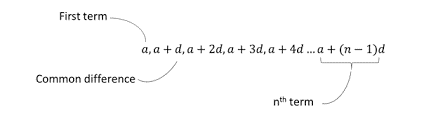The first term of the sequence is called the Initial Term which is denoted as ‘a’.

The difference by which the consecutive numbers of the sequence increases or decreases is called the Common Difference, which is denoted by‘d’.

The general term that is, the nth term of the arithmetic progression with the initial term a and the common difference d is as

an = a + (n-1) d

Example

Consider the right figure-Here the sequence is given as

1, 3, 5, 7, 9, 11, 13, 15

Given a = 1 and d = 2

Let’s check the value of the 5th term using the nth term formula.

an = a + (n-1) d

a5 = 1 + (5 -1)2

= 1 + 8

= 9

It is given in the right figure that the 5th term is 9.

Finite and Infinite Arithmetic Progression

The arithmetic progression with a limited number of terms is called Finite Sequence. It has a last term.

Example

2, 9, 16, 23,…, 86

This is a finite sequence with a = 2 and d = 7

The arithmetic progression with unlimited number of terms is called Infinite Sequence. It does not have a last term.

Example

1, 3, 5, 7, …

This is an infinite sequence with a = 1 and d = 2

Positive and Negative Common Difference

It depends upon the value of the common difference that the arithmetic progression is increasing or decreasing.

If the common difference ‘d’ is positive then the arithmetic progression will increase towards positive infinity that is, it will be an increasing sequence.

If d > 0 and satisfies that an-1 < an , then

​a1 < a2 < a3…

Example

11, 22, 33, 44, 55, …

This is an increasing sequence with a = 11 and d = 11.

If the common difference ‘d’ is negative then the arithmetic progression will rise towards negative infinity that is, it will be a decreasing sequence.

If d < 0 and satisfies that an-1 > an , then

​a1 > a2 > a3…

Example

20, 10, 0, -10, -20, …

This is a decreasing sequence with a = 20 and d = -10.

Properties of Arithmetic Progression

If the terms of the arithmetic progression are increased or decreased with the same number then the resultant sequence will also be an arithmetic progression.

Example

3, 6, 9,… is an AP with a = 3 and d = 3

If we will add 2 to every term of the sequence then the new sequence will be

5, 8, 11, … with a = 5 and d = 3

Hence, this is also an arithmetic progression.

If the terms of the arithmetic progression are multiplied or divide with the same number (non-zero) then the resultant sequence will also be an arithmetic progression.

Example

1, 2, 3, 4,… is an AP with a = 1 and d = 1

If we multiply the every term of the sequence with 5 then the new sequence will be

5, 10, 15, 20, … with a = 5 and d = 5

Hence, this is also an arithmetic sequence.

Graphical Representation of the Arithmetic Progression

The Arithmetic Progression can be shown through the graphs also. It could be on number line or on the Cartesian plane.

Representation on Number Line

On the number line, the AP can be shown as follows: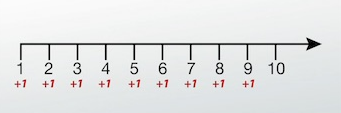Here in the above number line, it is showing the arithmetic progression

1, 2, 3, 4, 5, 6, …

with a = 1 and d = +1

Representation on  the Cartesian Plane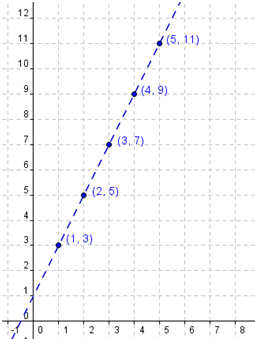AP = 3, 5, 7, 9, 11

a = 3 and d = +2

Here we need the Cartesian coordinates to represent it on the Cartesian plane.

an is the nth term, so the corresponding Cartesian coordinates will be in the form of (n, an ).

For the right arithmetic progression the coordinates will be as follows:

Arithmetic Series

When we add the terms of the arithmetic progression then that sum is the arithmetic series. Like, the AP, the difference between every consecutive term is always constant.

The arithmetic series is in the form of

{a + (a + d) + (a + 2d) + (a + 3d) + .........}

where a is the first term of the series and d is the difference of it which is known as the common difference of the given series.

Formula of nth term of Arithmetic Series

If a is the first term, d is the difference and n is the total number of the terms, then the formula for nth term is given by

(1, 3), (2, 5), (3, 7), (4, 9), (5, 11)

an = a + (n - 1) d

Example

What is the 10th term of the series 2 + 4 + 6 + 8 +…?

Solution:

In the given series a = 2 and d = 2

So we will put the values in the formula of nth term of the series.

an = a + (n - 1) d

Here n = 10 as we have to find the 10th term.

a10 = 2 + (10 - 1) 2

= 2 + 18

= 20

So the 10th term of the series will be 20

Sum of an Arithmetic Series

If a is the first term and d is the common difference of the series with n number of terms, then the sum of the series will be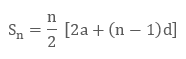Example

Find the sum of the given arithmetic progression

2 + 5 + 8 + 11 + 14

Solution:

Given

a = 2 (first term)

d = 3 (common difference)

n = 5 (as the total number of terms of the series is 5)

Now we will put the values in the formulaIf we know the last term ‘l’ of the series instead of the common difference ‘d’, then the sum of the series would beLet’s take the same example as above

2 + 5 + 8 + 11 + 14

Now we will find the sum of the series with a= 2 and l = 14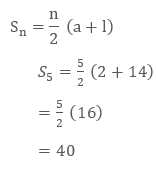Hence the sum remains the same if we calculate with the formula.

Arithmetic Mean

Arithmetic Mean is basically the average of two numbers. If we have two numbers n and m, then we can include a number L in between these numbers so that the three numbers will form an arithmetic sequence like n, L, m.

In that case the number L is the arithmetic mean of the numbers n and m.

According to the property of Arithmetic progression, we can say that-

L – n = m – L  that is, the common difference of the given AP.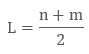This is generally used to find the missing number of the sequence between the two given numbers.

Example

What will be the 6th number of the sequence if the 5th term is 12 and the 7th term is 24?

As the two numbers are given so the 6th number will be the Arithmetic mean of the two given numbers.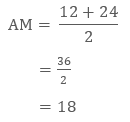Hence the 6th term will be 18.

We can insert two or more numbers between the two given numbers to form an Arithmetic Progression.

Let A1 , A2 , A3 , …, An be n numbers between a and b such that  a, A1 , A2 , A3 , …,An , b is an A.P.

Here, b is the (n + 2) th term, that is, b = a + [(n + 2) – 1] d = a + (n + 1) d.

This givesThus, n numbers between a and b are as follows:Example

Insert 5 numbers between 3 and 21 such that the resulting sequence is an A.P.

Solution:

Let A1, A2, A3, A4 and A5 be the five numbers between 3 and 21 such that

3, A1, A2, A3, A4, A5, 21

the sequence is in A.P.

Here, a = 3, b = 21, n = 7.

Therefore,

21 = 3 + (7 –1) d

18 = 6d

d = 3

Thus   A 1 = a + d = 3 + 3 = 6;

A 2 = a + 2d = 3 + 2 × 3 = 9;

A 3 = a + 3d = 3 + 3 × 3 = 12;

A 4 = a + 4d = 3 + 4 × 3 = 15;

A5 = a + 5d = 3 + 5 × 3 = 18;

Hence, five numbers between 3 and 21 are 6, 9, 12, 15 and 18

Arithmetic Progression (A.P.)
```### Course Features

• 731 Video Lectures
• Revision Notes
• Previous Year Papers
• Mind Map
• Study Planner
• NCERT Solutions
• Discussion Forum
• Test paper with Video Solution